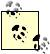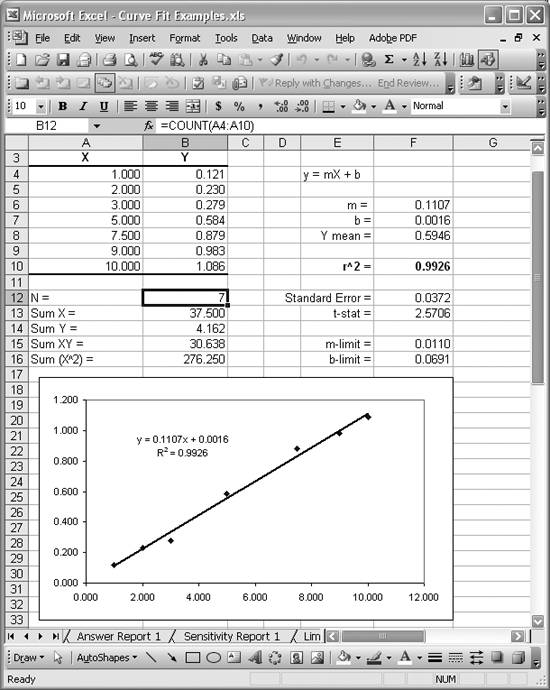# Computing Confidence Intervals

Problem

You want to compute confidence intervals along with your curve fits.

Solution

See the following discussion.

Discussion

You can compute confidence intervals for the estimated values from your curve fit, or you can compute confidence intervals for the parameters in the model itself.

Confidence intervals for estimated values

You can readily compute confidence intervals for the values predicted by a regression equation. In this case, you'd report estimated values as y ± yc, where y is the estimated value and each ±yc is the confidence limit corresponding to some degree of confidence (probability), c. To compute confidence limits, we'll utilize the Student's t-distribution , which comes from an area of statistics called small sampling theory . Excel provides support for the t-distribution through several built-in functions. See Recipe 5.6 for more information.

Let's reconsider the example from Recipe 8.6. Figure 8-12 shows the result of a nonlinear curve fit, along with upper and lower confidence limits. Figure 8-11 shows the original data along with the fit parameters. It also shows several other statistics I computed for this example. The ones of interest here are t-value and Confidence Interval, shown in cells M13 and M14.

Before computing these values, you must set up some auxiliary calculations. First you must compute the sum of squared residuals as shown in cell M5. The formula used in this example is =SUMSQ(H5:H41), which is just the sum of the squares of the residuals already computed in column H.

Next you need to compute the residual standard deviation. This value is computed in cell M6 using the formula =SQRT(M5/M9), where the cell in the numerator is the sum of squared residuals and the cell in the denominator is the degrees of freedom for this example. Degrees of freedom is computed in cell M9 using the formula =M8-M7, which is the number of samples minus the number of parameters in the fit (7 in this case, for the seven b-parameters).

Now you can compute the t-statistic using the formula =TINV((1-M12), M9), the result of which is shown in cell M13. The function TINV returns the inverse of the Student's t-distribution corresponding to a specified probability. In this case, I set the degree of confidence to 95% (.95 probability) in cell M12 and used 1-M12 as the probability passed in as the first argument in TINV. The second argument to TINV represents the problem's degrees of freedom.

Finally, the confidence limits are found by multiplying the t-statistic by the residual standard deviation. Cell M14 contains this result, using the formula =M13*M6. To plot the upper and lower confidence limits on the same chart as the original data and fit curve, you must set up two new columns. The first column contains the estimated y-value plus the confidence limit, and the second column contains the estimated y-value minus the confidence limit. You can then add these two new series to your chart to obtain the results shown in Figure 8-12.

Confidence intervals for curve parameters

Sometimes it's the parameters of a curve fit that are of scientific interest, instead of the estimated values predicted from the fit line. For example, you may compute a best-fit straight line through a set of experimental data where the slope of the best-fit line represents some aspect of the underlying physical process, such as reaction rate or spring constant. Curve fitting to estimate the model parameters yields statistical estimates of those parameters given the sample, which we assume represents the entire population. We can use statistical techniques to estimate confidence intervals for these parameters just as we did when estimating confidence intervals for predicted values.

Figure 8-16 shows some experimental data that has been the subject of a linear least-squares curve fit. In this case, the parameters of the curve fit (the slope and intercept) are of scientific importance and we'll compute confidence limits for each of these corresponding to a 95% degree of confidence.

To begin with, you must fit a straight line through the data, using any of the linear curve-fitting techniques discussed in this chapter. Then you need to compute the standard error for the estimate by using Excel's built-in function STEYX. Cell F12 contains the formula =STEYX(B4:B10,A4:A10), which computes the standard error for a given set of y- and x-values. In this case, the y- and x-values are contained in columns B and A, respectively.STEYX computes the standard error assuming a linear fit. See Excel's help topic "STEYX" for more information.

The t-statistic is computed in a manner similar to that discussed earlier. In this case, cell F13 computes the t-statistic using the formula =TINV(0.05,(B12-2)).

Now you need to set up a column computing the x - xmean for each sample. These aren't shown in Figure 8-16, but all you need to do is set up another column containing the formula =A4-AVERAGE(\$A\$4:\$A\$10) for each x-value contained in cells A4 through A10. Once that's set up, you can compute the sum of squares of the values in that column by using the formula =SUMSQ(L4:L10). In my spreadsheet, I put the x - xmean calculations in the cell range L4:L10.

Finally, the confidence limits for the slope and intercept are computed in cells F15 and F16, respectively. Cell F15 contains the formula =F13*F12/SQRT(L11), which

Figure 8-16. Parameter confidence intervals exampleyields a confidence limit of ± 0.0110 for the slope. Cell F16 contains the formula =F13*F12*SQRT(1/B12+AVERAGE(A4:A10)^2/L11), which yields a confidence limit of ± 0.0691 for the intercept.

The results of this exercise would be reported as follows: slope m = 0.1107 ± 0.0110; intercept b = 0.0016 ± 0.0691.

See Recipes 5.3 and 5.6 for more information on Excel's support for the Student's t-distribution.Excel Scientific and Engineering Cookbook (Cookbooks (OReilly))
ISBN: 0596008791
EAN: 2147483647
Year: N/A
Pages: 206
Authors: David M Bourg